# 09-240/Classnotes for Thursday September 10

Jump to: navigation, search
WARNING: The notes below, written for students and by students, are provided "as is", with absolutely no warranty. They can not be assumed to be complete, correct, reliable or relevant. If you don't like them, don't read them. It is a bad idea to stop taking your own notes thinking that these notes can be a total replacement - there's nothing like one's own handwriting! Visit this pages' history tab to see who added what and when.

## Date: Thurs. Sept. 10, 2009

• Professor's name: Dror Bar-Natan
• Solve systems of equations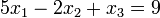$5x_{1} - 2x_{2} + x_{3} = 9$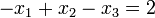$-x_{1} + x_{2} - x_{3} = 2$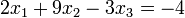$2x_{1} + 9x_{2} - 3x_{3} = -4$

• how? when? one/many?
• This describes the small-scale behaviour of almost everything that has a mathematical description.

1. A matrix is a square or rectangular array of numbers.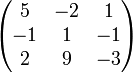$\begin{pmatrix} 5 & -2 & 1\\ -1 & 1 & -1\\ 2 & 9 & -3 \end{pmatrix}$
• we will learn addition, multiplication, and powers of matrices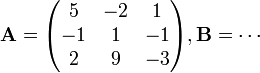$\mathbf{A}=\begin{pmatrix} 5 & -2 & 1\\ -1 & 1 & -1\\ 2 & 9 & -3 \end{pmatrix}, \mathbf{B}=\cdots$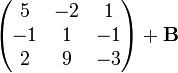$\begin{pmatrix} 5 & -2 & 1\\ -1 & 1 & -1\\ 2 & 9 & -3 \end{pmatrix}+\mathbf{B}$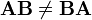$\mathbf{AB} \neq \mathbf{BA}$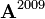$\mathbf{A}^{2009}$

• describes the approximate long-term behaviour of almost anything...
• Do all this without choosing coordinates.

2. Do everything over other “systems of numbers”

1. real numbers
2. rational numbers
3. complex numbers (things like alternating current, circuit)
4. {0,1} (binary, computer science)

3. Hidden Agenda

• Learn the basic pure-math processes of: abstraction, generalizations, definitions, theorems, proofs, notation logic

4. Administration

• can add things to wiki (so long as relevant to course material)
• any page added to wiki must start with 09-240- or 09-240/
• HW assigned on Tuesday, due in tutorial 9 days later.
• HW graded and returned by following tutorial

5. Classwork done today

• The Real Numbers: a set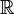$\mathbb{R}$ with two binary operations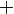$\,\!+$,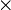$\times$(2 inputs, one output) and also with two distinguished elements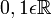$0,1\epsilon\mathbb{R}$ with the following properties:

R1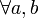$\forall a,b$

1.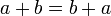$\,\!a + b = b + a$
2.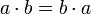$a \cdot b=b \cdot a$

Aside: The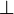$\perp$ character used for additon:

• Prof. Dror asked why$+$ is sometimes written as$\perp$?
• This is a Jewish tradition that dates back to at least the 19th century, and is still used today in Israeli elementary schools. It avoids the writing of the$+$ symbol, which resembles a Christian cross. (reference: http://en.wikipedia.org/wiki/Plus_and_minus_signs#Alternative_plus_sign)